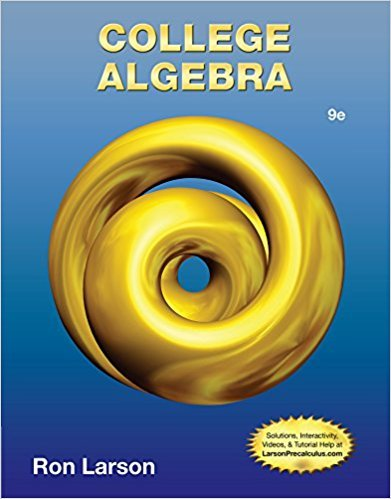×
×

# An ordered pair is a ________ of an equation in and whenISBN: 9781133963028 204

## Solution for problem 1.1.1 Chapter 1

College Algebra | 9th Edition

• Textbook Solutions
• 2901 Step-by-step solutions solved by professors and subject experts
• Get 24/7 help from StudySoup virtual teaching assistantsCollege Algebra | 9th Edition

4 5 0 248 Reviews
29
3
Problem 1.1.1

An ordered pair is a ________ of an equation in and when the substitutions and result in a true statement.

Step-by-Step Solution:
Step 1 of 3
Step 2 of 3

Step 3 of 3

##### ISBN: 9781133963028

This textbook survival guide was created for the textbook: College Algebra, edition: 9. This full solution covers the following key subjects: . This expansive textbook survival guide covers 9 chapters, and 5750 solutions. The answer to “An ordered pair is a ________ of an equation in and when the substitutions and result in a true statement.” is broken down into a number of easy to follow steps, and 20 words. Since the solution to 1.1.1 from 1 chapter was answered, more than 251 students have viewed the full step-by-step answer. The full step-by-step solution to problem: 1.1.1 from chapter: 1 was answered by , our top Math solution expert on 01/02/18, 09:21PM. College Algebra was written by and is associated to the ISBN: 9781133963028.

Unlock Textbook Solution